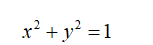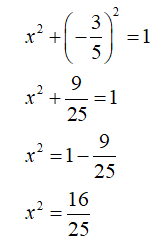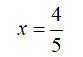# The point P is on the unit circle. Find P(x, y) from the given information.The y-coordinate of P is −3/5, and the x-coordinate is positive.

Question
1 views

The point P is on the unit circle. Find P(xy) from the given information.

The y-coordinate of P is
−3/5,
and the x-coordinate is positive.
check_circle

Step 1

Since the point P(x,y) is on the unit circle ,so it satisfies the following equationStep 2

Now, we will substitute y=-3/5 and solve for xSince x is positive ,therefore...

### Want to see the full answer?

See Solution

#### Want to see this answer and more?

Solutions are written by subject experts who are available 24/7. Questions are typically answered within 1 hour.*

See Solution
*Response times may vary by subject and question.
Tagged in

### Trigonometry